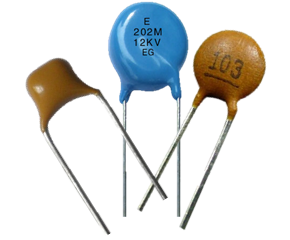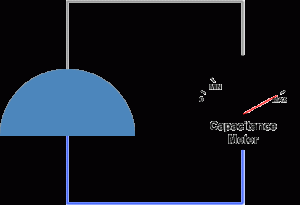What are ceramic capacitors? A ceramic capacitor uses a ceramic material as the dielectric. Ceramics were one of the first materials to be used in the producion of capacitors, as it was a known insulator. Many geometries were used in ceramic capacitors, of which some, like ceramic tubular capacitors and barrier layer capacitors are obsolete today due to their size, parasitic effects or electrical characteristics. The types of ceramic capacitors most often used in modern electronics are the multi-layer ceramic capacitor, otherwise named ceramic multi-layer chip capacitor (MLCC) and the ceramic disc capacitor. MLCCs are the most produced capacitors with a quantity of approximately 1000 billion devices per year. They are made in SMD (surface-mounted) technology and are widely used due to their small size. Ceramic capacitors are usually made with very small capacitance values, typically between 1nF and 1µF, although values up to 100µF are possible. Ceramic capacitors are also very small in size and have a low maximum rated voltage. They are not polarized, which means that they may be safely connected to an AC source. Ceramic capacitors have a great frequency response due to low parasitic effects such as resistance or inductance. Ceramic capacitor definition A ceramic capacitor is a capacitor which uses a ceramic material as the dielectric. The two most common types are multi-layer ceramic capacitors and ceramic disc capacitors. Characteristics Precision and tolerances There are two classes of ceramic capacitors available today: class 1 and class 2. Class 1 ceramic capacitors are used where high stability and low losses are required. They are very accurate and the capacitance value is stable in regard to applied voltage, temperature and frequency. The NP0 series of capacitors has a capacitance thermal stability of  ±0.54% within the total temperature range of -55 to +125 °C. Tolerances of the nominal capacitance [… read more]What are air capacitors? Air capacitors are capacitors which use air as their dielectric. The simplest air capacitors are made of two conductive plates separated by an air gap. Air capacitors can be made in a variable or fixed capacitance form. Fixed capacitance air capacitors are rarely used since there are many other types with superior characteristics. Variable air capacitors are used more often because of their simple construction. They are usually made of two sets of semicircular metal plates separated by air gaps. One set is fixed and the other is attached to a shaft which allows the user to rotate the assembly, therefore changing the capacitance as needed. The larger the overlap between the two sets of plates, the higher the capacitance. The maximum capacitance state is achieved when the overlap between the two sets of plates is highest, while the lowest capacitance state is achieved when there is no overlap. Variable air capacitors are used in circumstances where the capacitance needs to be varied. They are sometimes used in resonant circuits, such as radio tuners, frequency mixers or antenna impedance matching applications. Another use for variable capacitors is while prototyping an electronic circuit design. Sometimes, it is simply easier to find the appropriate capacitor value by trial and error instead of calculating it exactly. Air capacitor definition Air capacitors are capacitors which use air as the dielectric medium located between conductive plates. Characteristics The dielectric constant value of a material is a measure of the amount of electrical energy stored in a material for a given voltage. Since capacitors are devices used to store electrical energy, higher dielectric constants are favorable. However, the dielectric value of air is approximately that of vacuum, which is the lowest dielectric constant possible for a material and equals εo = 8.854 * 10-12 [… read more]